Author: Oscar Cronquist Article last updated on September 16, 2022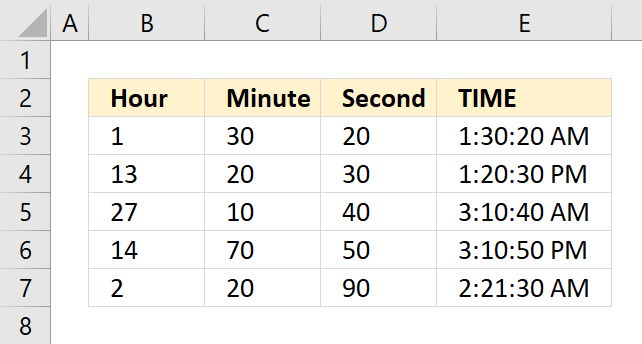The TIME function returns a decimal value between 0 (zero) representing 12:00:00 AM and 0.99988426 representing 11:59:59 P.M.

Formula in cell D3:

=TIME(B3, C3, D3)

## 1. TIME Function Syntax

TIME(hour, minute, second)

## 2. TIME Function Arguments

 hour Required. A number between 0 and 32767 represents the hour. minute Required. A number between 0 and 32767 represents the minute. second Required. A number between 0 and 32767 represents the second.

## 3. What is time in Excel?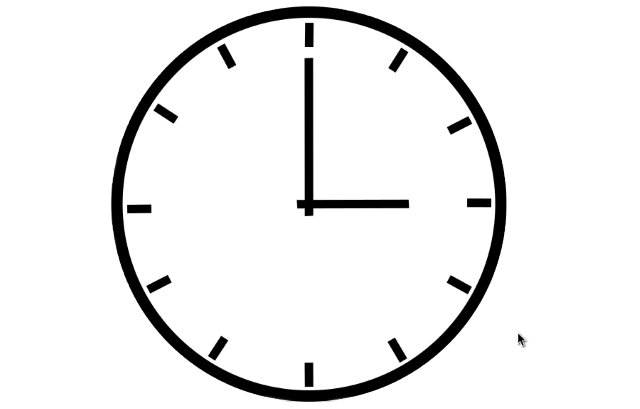Excel time value is a number equal to or larger than 0 (zero) and smaller than 1, formatted as a time value. One hour is 1/24, there are 24 hours in one day.

One minute is 1/1440, there are 1440 minutes in one day (60*24 = 1440). One second is 1/86400, there are 86400 seconds in one day (60*60*24 = 86400).

The following table shows whole hours, one hour is 1/24, 2 hours is 2/24, and so on.

0 - 12:00:00 AM
1/24 - 1:00:00 AM
2/24 - 2:00:00 AM
...
23/24 - 11:00:00 PM
24/24 - 12:00:00 AM

The time value is only the decimal part of a number, in other words, a value larger than or equal to 1 makes no difference, Excel uses only the decimal part of a number to create an Excel time value.

1.5 -> 0.5 -> 12:00:00 PM

The whole numbers represent dates in Excel. The whole number and the decimal part create a date and time value. Here is an example: 1.5 represents 1/1/1900 12:00 PM

## 4. TIME Function exampleThe TIME function creates an Excel time value meaning a number equal to or larger than 0 (zero) and smaller than 1 formatted as a time value.

Formula in cell E3:

=TIME(B3, C3, D3)

### Explaining formula

#### Step 1 - TIME function

TIME(hour, minute, second)

#### Step 2 - Populate arguments

TIME(hour, minute, second)

hour - B3
minute - C3
second - D3

#### Step 3 - Evaluate formula

TIME(1, 30, 20)

and returns 0.062731481 (1:30:20 AM).

1 hour = 1/24
30 min = 30/1440
20 sec = 20/86400

1/24 + 30/1440 + 20/86400 = 0.062731481

### 4.1 Time function - Hour value larger than 24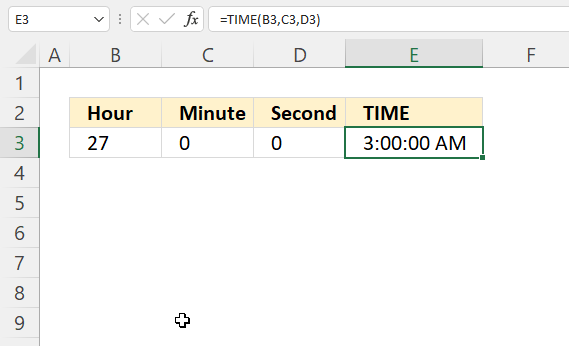An hour value greater than 23 will be divided by 24 and the remaining hours will be returned by the function.

27/24 = 1.125 The decimal part is 0.125 and is equal to 3 hours. 3/24 = 0.125

### 4.2 Time function - Minute value larger than 59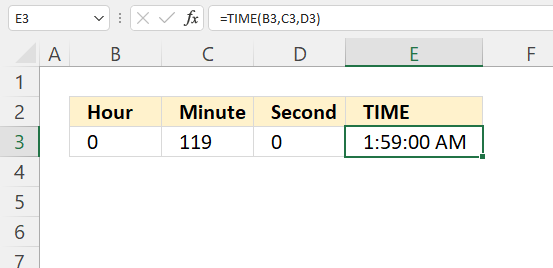A minute value equal to or greater than 60 will be divided by 60, the whole number is hours and the remaining minutes will be minutes.

119/60 is approx. 1.983333 The TIME function returns 1:59:00 AM. 0.983333 is approx. 59 minutes.

### 4.3 Time function - Seconds value larger than 59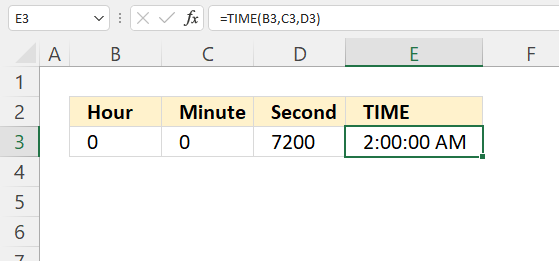A "second" value equal to or greater than 60 will be divided by 60 and added to minutes, the remaining seconds are returned.

7200/86400 is approx. 0.083333 which is the same as 120 minutes or 2 hours.

## 5. TIME Function - how to add hours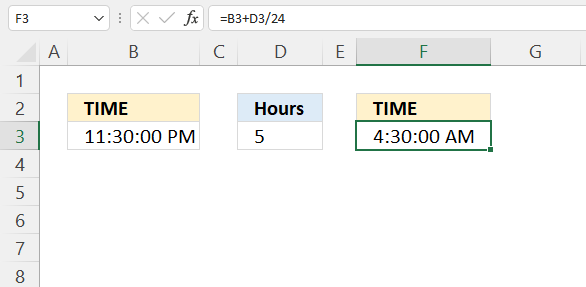Formula in cell F3:

=B3+D3/24

Alternative formula:

=TIME(HOUR(B3) + D3, MINUTE(B3), SECOND(B3))

Note, the TIME function arguments are limited to 32767. Larger values return #NUM errors.

### Explaining formula

#### Step 1 - Calculate hours in decimals

D3/24

becomes

5/24 equals 0.208333333.

#### Step 2 - Add time

B3+D3/24

becomes

0.979166667 + 5/24

becomes

0.979166667 + 0.208333333 equals 1.1875

## 6. TIME Function - how to add minutes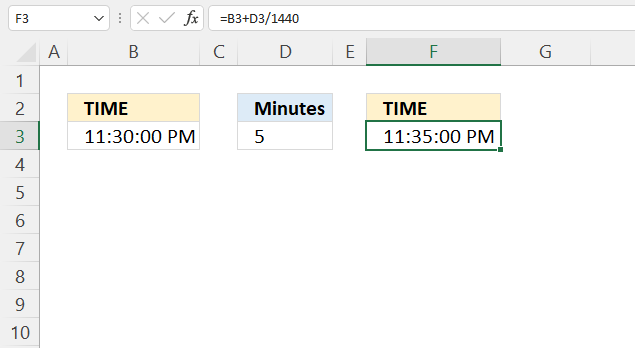Formula in cell F3:

=B3+D3/1440

Alternative formula:

=TIME(HOUR(B3), MINUTE(B3) + D3, SECOND(B3))

Note, the TIME function arguments are limited to 32767. Larger values return #NUM errors.

### Explaining formula

#### Step 1 - Calculate hours in decimals

The division slash charcater lets you divide numbers in an Excel formula.

D3/24

becomes

5/1440 is approx. 0.0034722

#### Step 2 - Add time

The plus sign lets you add numbers in an Excel formula.

B3+D3/1440

becomes

0.979166667 + 5/1440

becomes

0.979166667 + 0.0034722

and is approx. 0.982638889

## 7. TIME Function - how to add seconds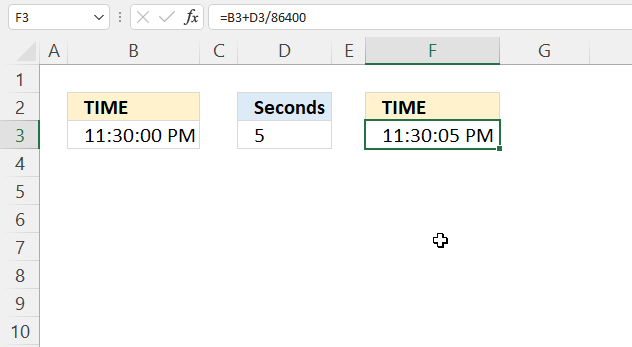Formula in cell F3:

=B3+D3/86400

Alternative formula:

=TIME(HOUR(B3), MINUTE(B3), SECOND(B3) + D3)

Note, the TIME function arguments are limited to 32767. Larger values return #NUM errors.

### Explaining formula

#### Step 1 - Calculate seconds in decimals

D3/24

becomes

5/86400

and is approx. 0.0000578

#### Step 2 - Add time

B3+D3/24

becomes

0.979166667 + 5/86400

becomes

0.979166667 + 0.0000578

and is approx. 0.979224537037037

## 8. How to calculate more than 24 hours?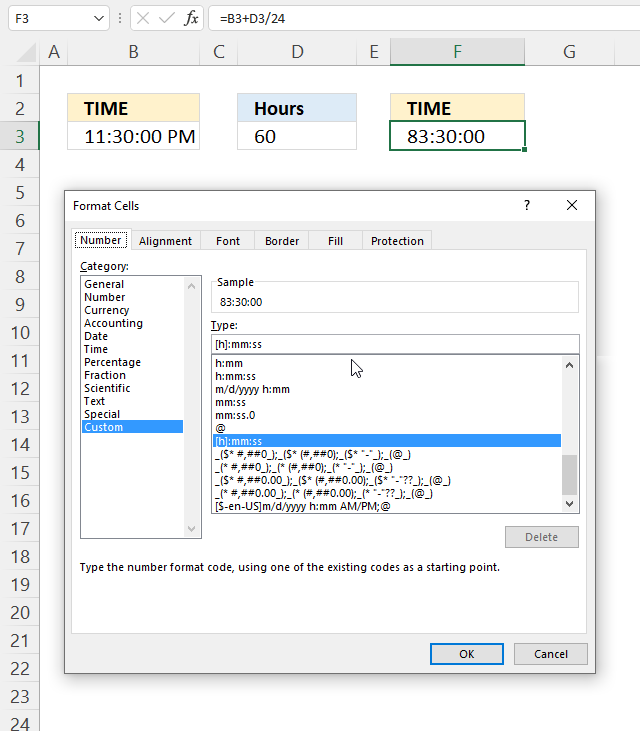The image above shows how to display an Excel time value larger than 1. This is possible using a different cell formatting code than the default one Excel uses.

1. Select cell F3.
2. Press CTRL + 1 to open the "Format Cells" dialog box.
3. Select the "Custom" category.
4. Use the following formatting code:
[h]:mm:ss
5. Press with left mouse button on OK button.

### Explaining formula in cell F3

#### Step 1 - Calculate Excel time value

Cell F3 contains an Excel time value larger than 1.

B3+B5/24

becomes

0.979166666666667 + B5/24

becomes

0.979166666666667 + 60/24

becomes

0.979166666666667 + 2.5

and returns 3.47916666666667 in cell F3. Cell F3 is formatted using the following cell formatting code: [h]:mm:ss which shows 83:30:00 in cell F3.

#### Step 2 - Verify calculation

We can easily verify the calculation.

3*24 = 72 hours

0.47916666666667 * 24 equals 11.5 hours.

72 + 11.5 = 83.5 hours -> 83:30:00

## 9. Convert time to 24 hour clock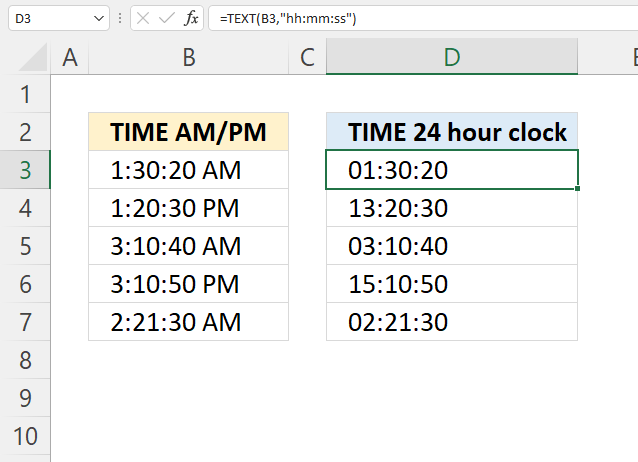The TEXT functionlets you convert AM/PM to a 24 hour clock.

Formula in cell D3:

=TEXT(B3,"hh:mm:ss")

### Explaining formula

#### Step 1 - TEXT function

The TEXT function converts a value to text in a specific number format.

TEXT(valueformat_text)

 value The string you want to format. You can use a cell reference here or use a text string. format_text Formatting code allows you to change the way, for example, a date or a number is displayed to the Excel user.

#### Step 2 - Populate TEXT function arguments

TEXT(valueformat_text)

value - B3

format_text - "hh:mm:ss"

hh - hours using two digits

mm - minutes (two digits)

ss - seconds (two digits)

#### Step 3 - Evaluate TEXT function

TEXT(B3,"hh:mm:ss")

becomes

TEXT(0.0627314814814815, "hh:mm:ss")

and returns 01:30:20.

1/24 + 30/1440 + 20/86400 equals 0.0627314814814815.